## Sunday, October 26, 2008

### The Shuffle

The shuffle, also known as the Fisher's Exact Test, is a permutation test that can be used to estimate the sampling distribution of a statistic without relying on parametric assumptions. This is especially important when sample sizes are small. The other neat thing about permutation tests is that you don't have to know what the distribution of your statistic is. So if you have a really odd function of your data that you want to use rather than one of the classical statistics you can.

Octave has a great built-in function called nchoosek which makes shuffling a breeze. Called with scalar arguments it returns the value of the binomial coefficient, which is the number of ways you can choose k things from n things (k<=n). For fixed n, nchoosek is maximum when k=n/2. That is plotted below for n=2:24.Notice that's a log-log scale, as n increases it quickly becomes intractable to perform a complete permutation test.

Suppose we have two data vectors, and we want to know if they are from populations with different means. We can use the normrnd() function to draw 8 samples from a normal distribution with 0 mean and 8 samples from a normal distribution with a mean of 1.

n=8;
x1 = normrnd(0,1,n,1);
x2 = normrnd(1,1,n,1);
perms = nchoosek( [x1;x2], n );

We stored all 12870 permutations of the vectors (16 choose 8) in the matrix perms. Now we use the built-in function complement to find the difference of the means under each of those labellings.

for(i=1:length(m1) )
comps(i,:) = complement( perms(i,:), [x1;x2] );
endfor
m1 = mean( perms, 2 ); % take row-wise averages, DIM=2
m2 = mean( comps, 2 ); % take row-wise averages, DIM=2
m_shuff = m1 - m2;

Now we can use the distribution of m_shuff to decide if the difference in the means of our two data vectors is significant.Octave script with all of these calculations: shuffle.m.

### Simple Bayes

I like Bayes theorem, it's really useful. The most intuitive and accessible explanation I've found of using Bayes theorem to solve a problem is in Russell and Norvig's classic, Chapter 20 (pdf) (I just own the first edition, the second edition looks even better).

The initial example they give is about pulling different flavoured candy out of a sack (remember the balls and urn from your basic stats?). They also provide a really good discussion showing how standard least-squares regression is a special case of maximum-likelihood for when the data are generated by a process with Gaussian noise of fixed variance.

Their first example is for estimating parameters in a discrete distribution of candy, but we can apply the same math to estimating the variance of a continuous distribution. Estimating variance is important, lots of times in industrial or business settings the variance of a thing matters as much or more than the average, just check-out all of the press those Six Sigma guys get. That's because it gives us insight into our risk. It helps us answer questions like, "What's our probability of success?" And maybe, if we're lucky, "What things is that probability sensitive to?"

Bayes theorem is a very simple equation:Where P(h) is the prior probability of the hypothesis, P(d|h) is the likelihood of the data given the hypothesis, and P(h|d) is the posterior probability of the hypothesis given the data.

Octave has plenty of useful built-in functions that make it easy to play around with some Bayesian estimation. We'll set up a prior distribution for what we believe our variance to be with chi2pdf(x,4), which gives us a Chi-squared distribution with 4 degrees of freedom. We can draw a random sample from a normal distribution with the normrnd() function, and we'll use 5 as our "true" variance. That way we can see how our Bayesian and our standard frequentist estimates of the variance converge on the right answer. The standard estimate of variance is just var(d), where d is the data vector.

The likelihood part of Bayes theorem is:

% likelihood( d | M ) = PI_i likelihood(d_i, M_j)
for j=1:length(x)
lklhd(j) = prod( normpdf( d(1:i), 0, sqrt( x(j) ) ) );
endfor
lklhd = lklhd/trapz(x,lklhd); % normalize it

Then the posterior distribution is:

% posterior( M | d ) = prior( M ) * likelihood( d | M )
post_p = prior_var .* lklhd;
post_p = post_p/trapz(x,post_p); % normalize it

Both of the estimates of the variance converge on the true answer as n approaches infinity. If you have a good prior, the Bayesian estimate is especially useful when n is small.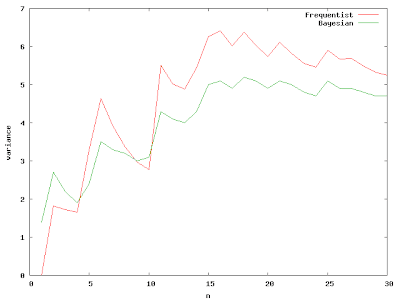It's interesting to watch how the posterior distribution changes as we add more samples from the true distribution.The great thing about Bayes theorem is that it provides a continuous bridge from what we think we know to reality. It allows us to build up evidence and describe our knowledge in a consistent way. It's based on the fundamentals of basic probability and was all set down in a few pages by a nonconformist Presbyterian minister and published after his death in 1763.

Octave file for the above calculations: simple_bayes.m

## Thursday, October 23, 2008

### Is Octave Slow?

I'm not going to talk about "computer time" vs. "programmer time"; though that's probably one of the most important considerations most of the time (check out Paul Graham's essays if you need convincing). I'll just talk about what takes a long time to compute in Octave, and what trade-offs can be made to improve the situation. We'll dwell mostly on the first tip from the Octave Manual (you'll see it's first for good reason). The other important thing to do is always allocate memory before looping over a vector (eg. x=zeros(n,1)).

Suppose we have a simple re-sampling problem. We would like to estimate the sampling distribution of a statistic of our data. The data could be a simple vector of 1 or 0 representing success or failure of a trial, and we want to estimate the probability of success.

x = [1,1,1,1,1,1,1,1,1,1,1,1,0,0,0,0];

One method to solve the problem would be to use a for loop:

X = empirical_rnd(N,x); % get a bootstrap sample from x
for(j=1:10) % timing loop, measure a couple times to get an idea of the
% variation
tic();
for( i=1:N-n ) % loop over the bootstrap samples
p(i) = sum( X(i:i+n-1) )/n;
endfor
fortime(j)=toc();
endfor

With N=20000, this takes 1.09 seconds on my old laptop, that seems kind of slow. The sampling distribution for the probability of success is shown in the figure below.It is always a good idea to vectorize what you can when in Octave, so the method below does just that.

X = empirical_rnd(x,N,n);
for(j=1:10)
tic();
P = sum(X,2)/n;
vecttime(j) = toc();
endfor

With n=20000, this takes 0.009 seconds on the same old laptop, that's a speed-up of 2 orders of magnitude!

Well, those are trivial examples of vectorizing a calculation to avoid the dreaded for-loop. What happens when subsequent calculations depend on the results of previous iterations?

tic();
p1(1) = 0;
for(i=2:N)
t = t + dt;
p1(i) = p1(i-1)+dt*2*t;
endfor
toc()

This loop integrates x*x numerically, with N=20000 it takes ~0.75 seconds. We can't vectorize, so how do we speed it up? Octave has some good sparse matrix capabilities, maybe we could recast the problem as a sparse matrix solve.Now we are trading memory for speed. In the for-loop implementation we just have to store the vector p. If we want to use Octave's sparse matrix facilities we need to store the two diagonals of our operator, so that roughly triples the memory requirements. Given the enormous size of modern computer memory, most toy problems should fit (if your problem doesn't fit, why are you still using Octave?).

tic();
A = spdiag( ones(N,1), 0 );
A = A + spdiag( -ones(N-1,1), -1 );
p2 = A\(dt*2*t);
toc()

The direct solution of the simple bi-diagonal system, with N=20000 takes ~0.019 seconds, better than 2 orders of magnitude speed-up over the for-loop implementation. For more complex sparse operators one of the iterative schemes might be appropriate.The moral: if your problem is small enough to fit into memory, cram it all in and don't use for-loops!

Is Octave slow? Well, it depends, if you use for-loops it is.

### Maxima Codes Fortran90 for Me

Maxima is great. In the magnetron post we integrated the differential equation describing an electron's motion in a couple of different ways. The implicit methods required a matrix inversion and then matrix-vector multiply for each time-step. Instead of writing out and coding the expressions describing that operation by hand (painful bugs quite likely, ouch!) it is much easier to let Maxima code the Fortran for us. If you have to ask "why Fortran?", then you are obviously some kind of quiche eating software weenie. If instead, you thought, "of course Fortran, what else would Maxima code?", please read on, you number crunching stud (or studette).

Start up a Maxima session (I run Fedora 9, but I'm sure most other distributions have it, I think they even have a windows installer). It's easy to define our matrix operator, invert it, and apply it:
L : matrix( [1,0,0, -dt, 0, 0], [0,1,0, 0, -dt, 0], [0,0,1, 0, 0, -dt], [0,0,0, 1, a*B/c,-a*B/c], [0,0,0,-a*B/c, 1, a*B/c], [0,0,0, a*B/c,-a*B/c, 1] );Linv : invert(L);X: [x,y,z,vx,vy,vz];P : a*[0,0,0,E,E,E];RHS : X - P;LinvRHS : factor( expand( Linv.RHS ) );

Simple as that we have a symbolic representation of our inverted operator acting on our state vector. Now we want Fortran90 expressions.
load("f90");fname : "back_euler_inv_op.f90";f : openw(fname);printf( f, "! These fortran expressions generated automatically from ! the maxima batch file magnetron.mac ~%");for i : 1 thru length(LinvRHS) do( printf( f, "x_n1(~d)=",i), with_stdout( f, f90(LinvRHS[i,1]) ) );close(f);

There is a small bug in the "f90" module (as of version 5.15) that causes it to use the Fortran77 style continuation when writing out a matrix, so that is why each element must be written out with a separate call to f90().

Well, that's neat, but I need to appease the quiche eaters, so wouldn't it be better if these expressions, and our inner loops (the time integration loop for this case) in Fortran were callable from something flexible and trendy like Python? That's where F2Py comes in. Generating a module for Python is one simple call:
f2py -c back_euler_sub.f90 -m back_euler
Where my subroutine using the Maxima generated expressions is in the file "back_euler_sub.f90" and the module will be named "back_euler".

The python script to use our new module is quite short:
from numpy import *from back_euler import *B = zeros( 3 )E = zeros( 3 )x_n = zeros( 6 )x_n1 = zeros( 6 )q = -4.8032e-10m = 9.1094e-28c = 2.9979e10dt = 3e-10nt = 50E = -1.0/3.0B = 33.6412X = zeros( (6,nt), dtype='double', order='F' )X = back_euler(nt,dt,q,m,c,E,B)
Now our low-level number crunching and inner loop is in nice, fast compiled F90 and we can call it from a high-level, object-oriented language to do things like zero finding (as in the magnetron post), or optimization or something else entirely.

## Wednesday, October 22, 2008

Since Google Reader went away I lost the easy way to share interesting articles. This post is a place to drop comments with links to interesting articles and things that don't rise to the level of a full post.

### Planar Magnetron

A magnetron is a device useful for generating lots of microwave radiation, most homes have magnetron's which use roughly 1kW of microwave power for heating food. A magnetron acts like a diode, when the magnetic field between the inner cathode and the outer anode reaches a certain level, electrons starting at the cathode will not be able to reach the anode. This level of magnetic field is known as the Hull cut-off magnetic field of the magnetron.

Suppose we have a simple planar magnetron with constant E and B fields. The path taken by an electron starting from rest at the cathode is governed by the relation of the time rate of change of the particle to the forces on the particle caused by the E and B fields.Assuming constant particle mass and neglecting relativistic effects gives an equation for particle acceleration:This second order differential equation can be recast as a system of first order equations: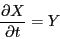Where X is the vector of position and velocity components given byAnd Y is the vector of velocity and acceleration components given byThe initial conditions are X = 0 andThis system can be integrated numerically with the explicit forward Euler method:The bisection method is used to find the value of B that causes a maximum of 1 cm
displacement in the y-direction. This 1 cm-high path taken by the electron with Bz = 33.7884
Gauss and Ey = −1/3 stat-volt/cm is shown in the figure below.
Explicit Integration:Alternatively, an implicit backward Euler method can be used: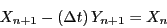The left hand side can be consolidated into a single operator, L, acting on the unkown vector at the future time-step and the additive term due to the electric field: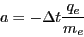This operator must be inverted, this can be done once and then each successive time-step
can be calculated with a matrix-vector multiply.First Order Implicit Integration:Using a second order accurate approximation for the first derivative (see finite difference for their relationship with derivatives) provides a more accurate solution, and makes the solution for the larger time-step sizes more reasonable:Collecting all of the n + 1 terms as we did before, givesOur implicit integration operator then just has a few slight modifications, and the right hand side now includes n and n − 1 terms.Second Order Implicit Integration:The second order method achieves something closer the "correct" shape much more quickly than the first-order scheme.

This analysis ignores any losses due to radiation from the accelerated particle (which of course is why magnetrons are useful in the first place).

A pdf and various Octave/Python/Maxima scripts for this problem are on:

### Current in the middle of the Earth

What if the Earth's magnetic field were caused by a current loop in the mantle?

This can be a decent approximation because the Earth's magnetic field is roughly that of a dipole.

It's easy to look-up the Radius of the earth, and we can use a rough estimate of 0.23 gauss for the horizontal component of the magnetic field at 40 degrees latitude.

A simple expression for the magnetic field due to a dipole in spherical polar coordinates is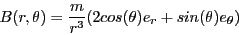There's no dependence on azimuthal angle because of the symmetry axis along the dipole.

The dipole moment is thenAnd the current in a loop 1/3 the radius of the earth required to create a field of that strength isThat's a lot of statcoulombs!

This is Problem 2.2 from Classical Electromagnetic Radiation; Chapter 2 covers multi-pole expansions of static electric and magnetic fields.

### First Post!

I never get to do that on Slashdot, so here it is.

This is a test post to play around with the Blogger stuff.